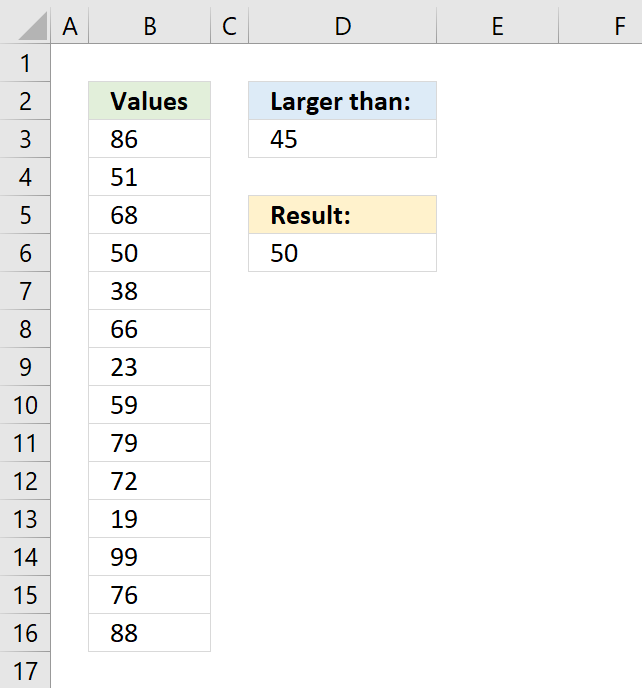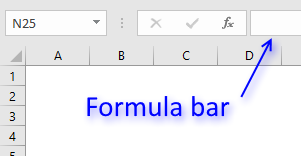Author: Oscar Cronquist Article last updated on January 20, 2023This article demonstrates formulas that lets you extract the smallest number larger than a given number.

The example above specifies the given number in cell D3, in this case, 45. The data is in cell range B3:B16, the formula in cell D6 calculates the smallest value but larger than 45.

## 1. Find the smallest number in a list that is larger than a given number - [Excel 2016]Formula in cell D6:

=MINIFS(B3:B16,B3:B16,">"&D3)

### 1.1 Explaining formula

#### Step 1 - Populate arguments

The MINIFS function calculates the smallest value based on a given set of criteria.

Function syntax: MINIFS(min_range, criteria_range1, criteria1, [criteria_range2, criteria2], ...)

MINIFS(min_range, criteria_range1, criteria1, [criteria_range2, criteria2], ...)

becomes

MINIFS(B3:B16,B3:B16,">"&D3)

The greater than sign > is a logical operator that lets you check if a number is larger than another number.

The ampersand character & lets you concatenate values in an Excel formula. You also have the option to enter the greater than sign in cell D3 combined with the given number, like this: >45. If you do remove the less than sign and the ampersand and reference only cell D3 in the MINIFS function.

#### Step 2 - Evaluate MINIFS function

MINIFS(B3:B16,B3:B16,">"&D3)

becomes

MINIFS({86; 51; 68; 50; 38; 66; 23; 59; 79; 72; 19; 99; 76; 88},{86; 51; 68; 50; 38; 66; 23; 59; 79; 72; 19; 99; 76; 88},">"&45)

and returns 50.

## 2. Find the smallest number in a list that is larger than a number - earlier Excel versionsArray formula in cell D6:

=MIN(IF(B3:B16>D3,B3:B16))

### 2.1 How to enter an array formula

1. Type the formula in cell B3
2. Then press and hold CTRL and SHIFT simultaneously,
3. Now press Enter once.
4. Release all keys.The formula bar now shows the formula with a beginning and ending curly bracket telling you that you entered the formula successfully.

Don't enter the curly brackets yourself, they appear automatically.

### Explaining array formula

#### Step 1 - Filter values in cell range larger than the condition in cell D3

The IF function allows you to create a logical expression that evaluates to TRUE or FALSE. You can then choose what will happen to a value that returns TRUE and also FALSE.

IF(logical_test, [value_if_true], [value_if_false])

If you use a cell range instead of a cell reference to a single cell you evaluate all the values in the cell range. The larger than sign lets you check if the values in B3:B16 are larger than the value in D3.

IF(B3:B16>D3,B3:B16)

becomes

IF({86;51;68;50;38;66;23;59;79;72;19;99;76;88}>45,B3:B16)

becomes

IF({TRUE; TRUE; TRUE; TRUE; FALSE; TRUE; FALSE; TRUE; TRUE; TRUE; FALSE; TRUE; TRUE; TRUE},B3:B16)

becomes

IF({TRUE; TRUE; TRUE; TRUE; FALSE; TRUE; FALSE; TRUE; TRUE; TRUE; FALSE; TRUE; TRUE; TRUE},{86; 51; 68; 50; 38; 66; 23; 59; 79; 72; 19; 99; 76; 88})

and returns the following array:

{86;51;68;50;FALSE;66;FALSE;59;79;72;FALSE;99;76;88}

#### Step 2 - Return the smallest value in the array

The MIN function ignores boolean values which is useful in this case.

MIN(IF(B3:B16>D3,B3:B16))

becomes

MIN({86;51;68;50;FALSE;66;FALSE;59;79;72;FALSE;99;76;88})

and returns 50 in cell D6.